# Matrix

Replenishment date: 01.05.2009
Content: matrix.rar (1.95 MB)
️Automatic issue of goods ✔️
Sales:
0
Refunds:
0
Reviews:
0
Views:
262Seller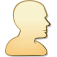Seller:
ORKus
Rating:
0
Report a violation
Description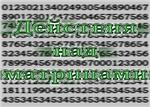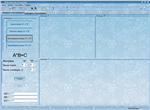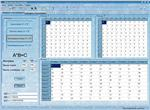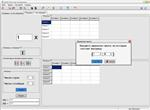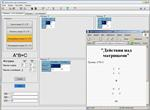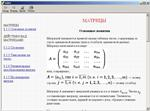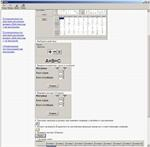The software product allows:
1. Set the sizes (number of rows and columns) of the processed matrices.
2. Fill in matrices with real numbers from the keyboard or randomly from the selected range.
3. Displaying filled matrices in natural form.
4. Perform arithmetic operations with one or two matrices.
5. Implement elementary matrix transformations:
a. permutation of two parallel rows of the matrix;
b. multiplication of all elements of the matrix row by a number other than zero;
c. adding to all the elements of the matrix row the corresponding elements of the parallel row, multiplied by the same number.
6. Perform matrix transposition.
7. Calculate the determinants of the processed matrices.
8. Save the results of matrix processing in HTML format.
9. Call the help system containing theoretical information on the "Matrix" section of higher algebra and the procedure for working with the application.
Similar items
Recommended